The following article is from The Great Soviet Encyclopedia (1979). It might be outdated or ideologically biased.

an expression of the form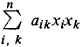(where aik = aki) that assumes nonnegative values for all real values of x1, x2, …, xn and that vanishes only when x1 = x2 = … = xn = 0. Thus, the positive definite quadratic form is a special case of a quadratic form. Any positive definite quadratic form can be reduced to the form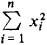by means of a linear transformation. In order for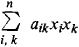to be a positive definite quadratic form, it is necessary and sufficient that Δ1 > 0…..Δn > 0, where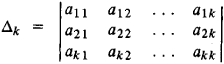In any affine coordinate system the distance of a point from the origin is expressed by a positive definite quadratic form in the coordinates of the point.

A Hermitian positive definite quadratic form is the form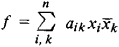such that aik = āki, f ≥ 0 for all values of x1, x2, …, xn, and f = 0 only when x1 = x2 = … = xn = 0; here overbar denotes the operation of complex conjugation.

The following concepts are also associated with positive definite quadratic forms: (1) positive definite matrix ǀǀaikǀǀn, which is a matrix such thatis a Hermitian positive definite form; (2) positive definite kernel, which is a function K(x,y) = K(y,x) such that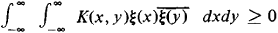for any function ξ(ξ) with integrable square; and (3) positive definite function, which is a function f(x) such that the kernel K(x,y,) = f(x — y) is positive definite. The class of continuous positive definite functions f(x) with f(0) = 1 coincides with the class of characteristic functions of the laws governing the distribution of random variables.

Mentioned in ?
References in periodicals archive ?
Solving such an inverse problem requires finding the potential energy of the system using data obtained from observations of the oscillations in it, they say, and because the oscillations are small, the potential energy is given by a positive definite quadratic form, the matrix of which is called the matrix of potential energy.
With the purpose to analyse the properties of the linear mapping (2.1), we may form a positive definite quadratic form function K(p, q) on E x E simply by taking
As about the possibilities in the product spaces, due to Schur's Theorem, we would like to recall that for any two positive definite quadratic form functions [K.sub.1](p, q) and [K.sub.2](p, q) on E x E, the usual product K(p, q) = [K.sub.1](p, q)[K.sub.2](p, q) is again a positive definite quadratic form function on E.
If [K.sub.1](p, q) and [K.sub.2](p, q) are two positive definite quadratic form functions on E, then the sum [K.sub.S](p, q) = [K.sub.1](p, q) + [K.sub.2](p, q) is also a positive definite quadratic form function on E.
Namely, in view to consider very general reproducing kernels represented by the Fourier integral, we define positive definite quadratic form functions [K.sub.j] (for j = 1, 2, 3) by
Hence, the image space that means the existence of the solution of (3.4) or (3.8) is characterized as the reproducing kernel Hilbert space admitting the positive definite quadratic form function
From condition A1, we get that [[summation].sup.r.sub.i=1] [d.sub.i][x.sup.T.sub.i][P.sub.i][x.sub.i] is a positive definite quadratic form, and, by the properties of f([sigma]), we know that V in (8) is radially unbounded, is positive definite, and has an infinitesimal upper bound.
are the components of a positive definite quadratic form on [R.sup.m], ,
[Mathematical Expression Omitted] is linearly spanned by theta series attached to quadratic forms in the genus of a specific totally positive definite quadratic form Q.
Computational geometry of positive definite quadratic forms; polyhedral reduction theories, algorithms, and applications.

Site: Follow: Share:
Open / Close